﻿ Common Core Standards for Math - KindergartenAdaptive Worksheets

Helping Every Child to Succeed

toll-free (888) 777-0876
fax (888) 777-0875

## Understand Place Value

2.NBT.1. Understand that the three digits of a three-digit number represent amounts of hundreds, tens, and ones; e.g., 706 equals 7 hundreds, 0 tens, and 6 ones. Understand the following as special cases:
• 100 can be thought of as a bundle of ten tens — called a “hundred.”
• The numbers 100, 200, 300, 400, 500, 600, 700, 800, 900 refer to one, two, three, four, five, six, seven, eight, or nine hundreds (and 0 tens and 0 ones).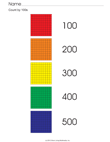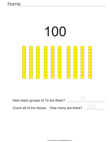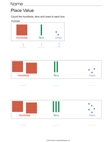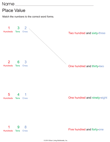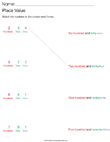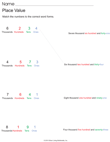2.NBT.2. Count within 1000; skip-count by 5s, 10s, and 100s.

2.NBT.3. Read and write numbers to 1000 using base-ten numerals, number names, and expanded form.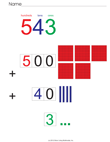Visual Representation of 3 Digit Numbers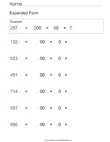Expanded Form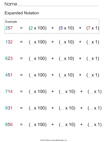Expanded Notation

2.NBT.4. Compare two three-digit numbers based on meanings of the hundreds, tens, and ones digits, using >, =, and < symbols to record the results of comparisons.

## Use place value understanding and properties of operations to add and subtract

• 2.NBT.5. Fluently add and subtract within 100 using strategies based on place value, properties of operations, and/or the relationship between addition and subtraction.Number Friends That Make Ten with Leaves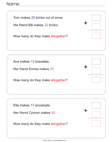Two Digit Addition Word Problems With Prompt Fading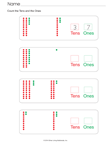Add Tens and OnesLeaf Addition Task Cards
• 2.NBT.6. Add up to four two-digit numbers using strategies based on place value and properties of operations.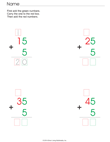Add a One Digit Number to a Two Digit Number with Regrouping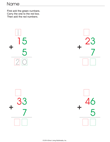Add a One Digit Number to a Two Digit Number with Regrouping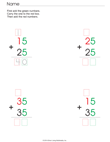Add Two Digit Numbers With Regrouping - Includes Visual Prompts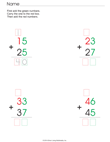Add Two Digit Numbers With Regrouping - Includes Visual Prompts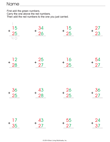Add Two Digit Numbers with Regrouping This set of worksheets has more problems per page and fewer prompts.
• 2.NBT.7. Add and subtract within 1000, using concrete models or drawings and strategies based on place value, properties of operations, and/or the relationship between addition and subtraction; relate the strategy to a written method. Understand that in adding or subtracting three-digit numbers, one adds or subtracts hundreds and hundreds, tens and tens, ones and ones; and sometimes it is necessary to compose or decompose tens or hundreds.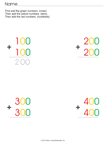Add Three Digit Numbers Without Regrouping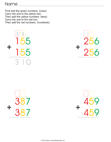Add Three Digit Numbers With Regrouping
• 2.NBT.8. Mentally add 10 or 100 to a given number 100–900, and mentally subtract 10 or 100 from a given number 100–900.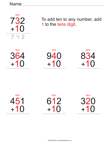Add Ten to 3 Digit Numbers  - Includes Visual Prompts
• 2.NBT.9. Explain why addition and subtraction strategies work, using place value and the properties of operations.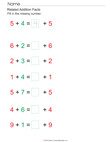Related Addition Facts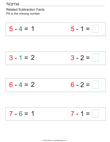Related Subtraction FactsFact Families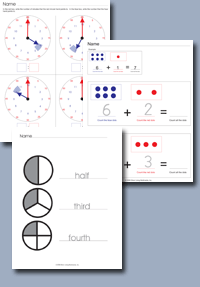Click on any of the standards on the left to create custom worksheets or to print premade materials.